# Introduction to Data Structure and Algorithm

## Introduction to Data Structure and Algorithm

In this article, I am going to give you a brief introduction to Data Structure and Algorithm. At the end of this article, you will understand what is Data Structure and Algorithm, its type, and why we need data structure and algorithm as well as Variables and Data Types.

##### Variables:

let’s take a simple mathematical equation.
x + y – 10 = 20 eq1.1
For now, we don’t need to worry about the equation. As we all can observe, the eq1.1 has some names which hold values(data). Similarly, in programming something required to hold data, and we called them variables. In eq1.1, x & y are variables that hold some data.

##### Data Types:

In eq1.1, x & y holds some data and the data may be integers or floats like 0,1, 0.5, -6.4 , -2 etc. A data type is a set of some predefined values like Integers, Floating points, character, strings, etc. There are two types of data types.

1. System – defined Data types (also called as Primitive data types)
2. User-defined Data types
##### System Defined Data Types

Primitive data type defined by the system. It is provided by programming language. Example – int, float, char, bool, etc. Allocation of bits for each primitive data type depends on programming language, operating system, and compiler. The different programming languages may allocate different sizes for the same primitive data type.

##### User-Defined Data Types

In many cases to solve a problem, system-defined data types are not enough. To overcome this situation many programming languages allow the users to define their own data type called user define data types.

Data Structures in C/C++ and classes in java are good examples. Generally, the user-defined data type is a combination of many primitive data types.

struct usrdef {
int sysdef1;
float sysdef2;
bool sysdef3;
};

##### What is Data Structure?

In computer science, a data structure is a data organization, management, and storage format that enables efficient access and modification. More precisely, a data structure is a collection of data values, the relationships among them, and the functions or operations that can be applied to the data.

In simple words, Data Structure is nothing but it is a particular way of organizing and storing data in a computer so that it can be accessed and modified in an efficient and easy way.

Let us understand the above definition with some examples. For example, if you have a scenario where you want to read the data sequentially as shown in the below image. Here, you can create a linked list data structure. In the Linked list data structure, the data points to the next data.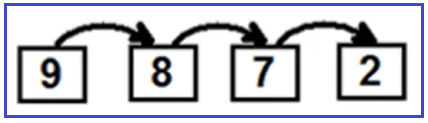Now another scenario where you need to display the organization structure where you have CEO, inside CEO, you have Manager and inside Manager, Technicians are there and inside technician, Helpers are there as shown in the below image. Then to represent such organization data, you need to go for Tree or graph data structure.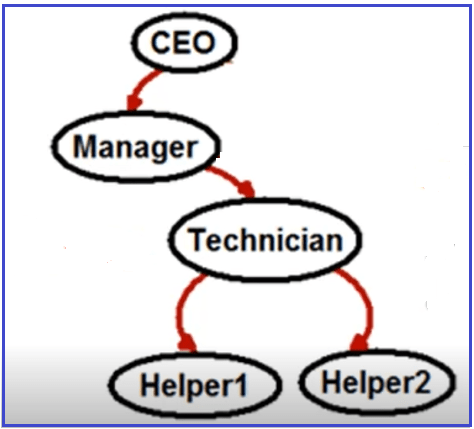Let say you have another scenario, where you want the data in a queue way. Queue means in the sequence the data is pushed into the data structure; in the same sequence, it should be retrieved from the data structure as shown in the below image. In such cases, you can create a Queue Data Structure or FIFO data structure.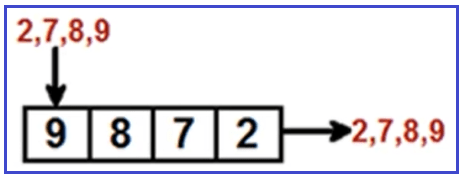So, Data Structure is nothing but it is all about organizing and storing data as per scenarios and needs so that it can be accessed and modified in an efficient and easy way.

##### Understanding Abstract Data Type (ADT):

In today’s world, there are more than 500 to 600 known data structures. Some of them are showing in the below diagram. Nothing about it, you have a situation and you have to go and choose the best data structure suitable for your needs.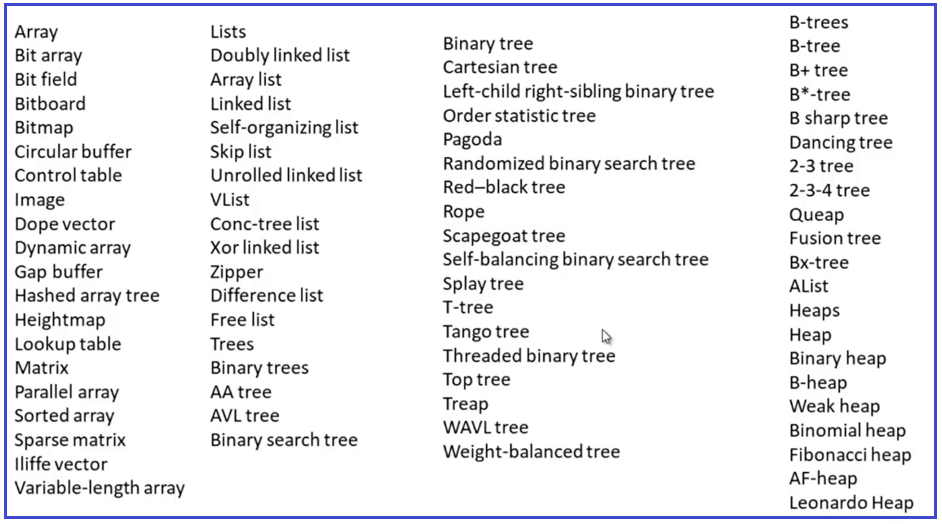And it’s really very difficult to remember each of these data structures. So, if you can go and categories them or you can think in an abstract way that would be great. For example, if have tress i.e. you can have a mango tree, you can have an orange tree, or you can have a coconut tree, and so on. If you think in an abstract way, this is just trees. They have roots, they have leaves and they have branches. For better understanding, please have a look at the below diagram.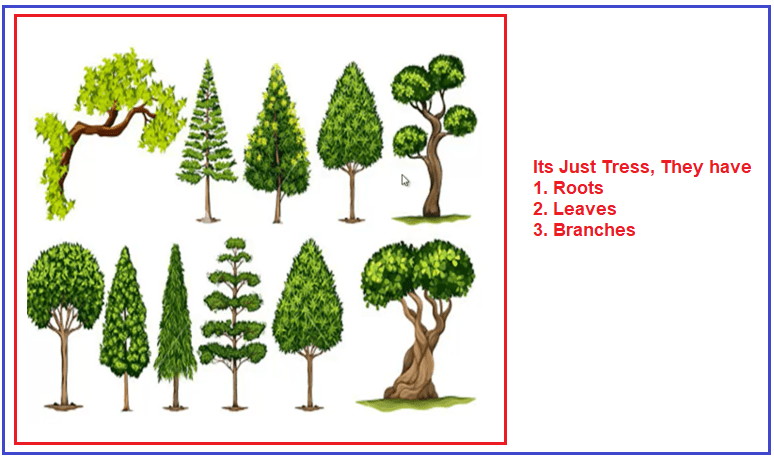In abstract thinking, we don’t have to go for each implementation and you know abstractly what you want to do. So, in a data structure point of view, instead of remembering all those 500 or 600 data structures, it would be great, if we group them into categories or abstract them into categories.

##### Example: Array

Let us think about the array. An array is one of the data structures. In array we have the following types:

1. One dimensional array
2. Two-dimensional array
3. Multi-Dimensional Array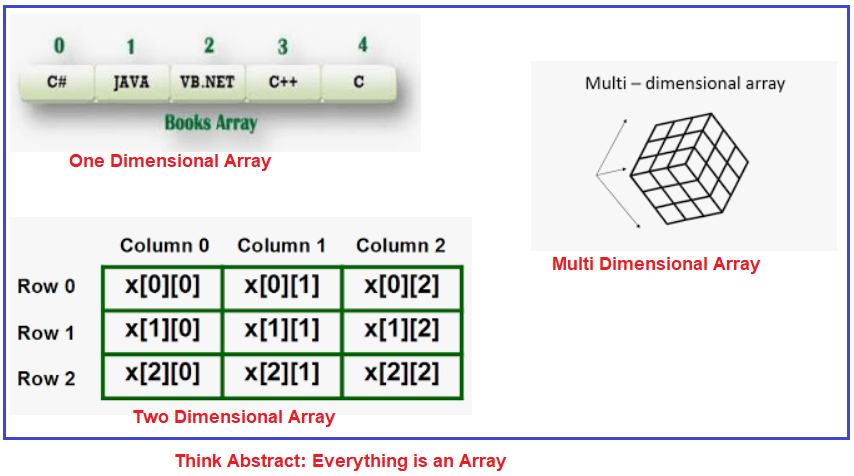Instead of remembering a one-dimension array, two-dimension array, multi-dimension array, think just abstract, it is an array. It can have values; it can have size. So, when you think about the abstract, then you don’t need to get into the implementation of each data structure.

Link List is another data structure. And we have Single, Double, and Circular Linked List. For better understanding please have a look at the below image.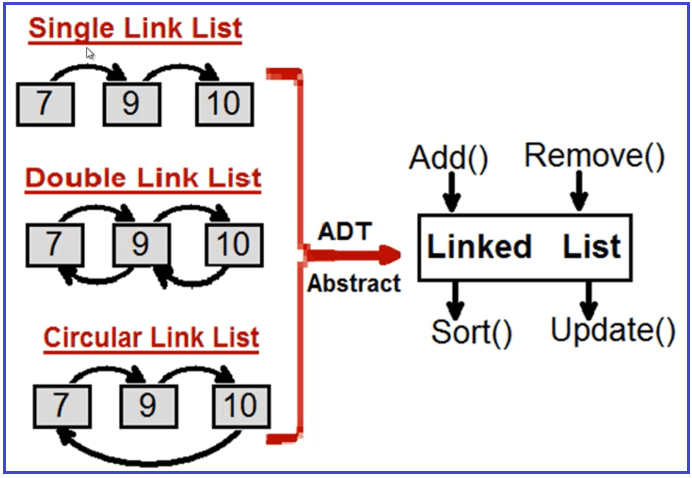So instead of remembering Single, Double, and Circular Linked List, think about in an abstract way. It is just a Linked List that has Add, Remove, Sort, and Update method.

So, if you think like this, then those 500 or 600 data structures just come under 5 or 6 ADT (Abstract Data Type).

##### Types of Data Structure:

Data Structure classified mainly into two types.

Linear Data Structure:- A Linear data structure have data elements arranged in a sequential manner and each member element is connected to its previous and next element. Example:- LinkedList, stacks, queue, trees, graphs, etc.

Non-Linear Data Structures:- A non-linear data structure has no set sequence of connecting all its elements and each element can have multiple paths to connect to other elements. Example:- Trees, Graphs.

For a better understanding of the classification of data structure please have a look at the following diagram.Key Differences between Linear and Non-Linear Data Structure##### Advantages of Data Structures or why we need Data Structure?

We need data structures, few of them are as follows:

1. They are essential ingredients in creating a fast and powerful algorithm.
2. They help to manage and organize data.
3. They make code cleaner and easier to understand.
##### Abstract Data Types

Basic operation like addition, multiplication, division, subtraction supported by primitive data type but for the user-defined data type, we need to define operations. such operations can be defined at the time of implementation.

In general, user-defined data types are defined along with their operations. The combination of data structures along with their operations is called Abstract Data Types (ADTs). It mainly consists of two parts.

1. Declaration of data
2. Declaration of operations

##### What is an Algorithm?

An algorithm is a step by step procedure or formula to solve a problem. And the step by step procedure is unambiguous. For example, you have a problem with sorting or you have a problem with doing some mathematical calculations. So, for that, there are time tested algorithms wherein the precise steps, the precise input, and the precise output are defined. And if you follow that algorithm, you will be able to solve that problem.

For example, if you want to add two numbers, the steps are crystal clear and there is no ambiguity. First, take number 1, then take number 3 and then add number1 and number 2 as shown in the below image. So, algorithms mean All go in a rhythm.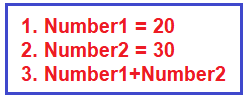##### Algorithms Characteristics:

Every code which we see in a project or every code which we write in a project does not classify as an algorithm. For example, if we write an insert operation or update operation or delete operation, it does not classify as an algorithm. To classify something as an algorithm, it has to satisfy five important characteristics.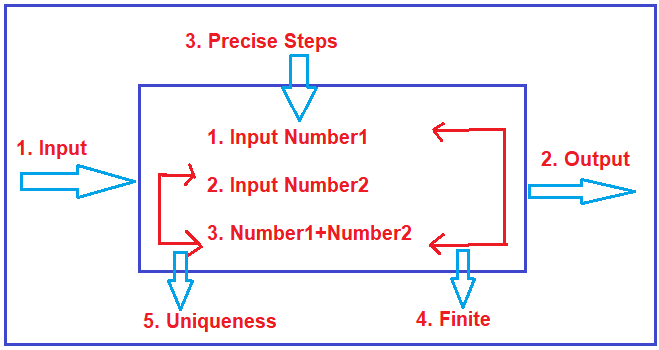First, there should be well-defined input and well-defined output. So, for an algorithm, the precise input and output should be crystal clear.

Next, the steps of the algorithms are precise, unambiguous, and to the points. For example, to add two numbers, the step should be first taking input number1 and then take input number2 and finally add number1 with number2. So, the steps for the algorithm are precise clear and it is finite.

Finite means this algorithm will start and will end after certain steps. It cannot just go in an infinite loop. Uniqueness means the output one step will go as an input into the next step. For example, the output of step1 i.e. Numbet1 must be used somewhere down below.

##### Properties of a good algorithm
1. Input and output should be clearly defined.
2. Each step in the algorithm should be clear and unambiguous.
3. Algorithms should be most effective among many different ways to solve a problem.

Note: The algorithm should be written in such a way that it can be used in different programming languages. No coding is allowed.

In the next article, I am going to discuss the Analysis of the Algorithm in detail. Here, in this article, I try to give a brief introduction to Data Structure and Algorithm and I hope you enjoy this Data Structure and Algorithm article. I hope you enjoy this Introduction to Data Structure and Algorithm article. I would like to have your feedback. Please post your feedback, question, or comments about this Data Structure and Algorithm article.